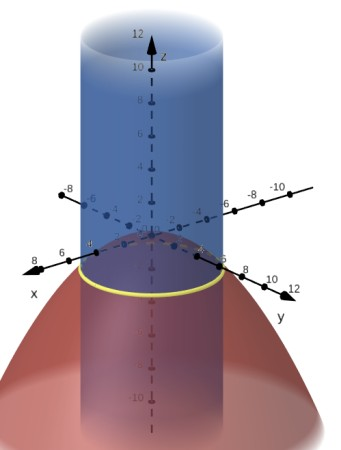# Find parametric equations that describe the curve defined by intersecting the cylinder r = 4 with...

## Question:

Find parametric equations that describe the curve defined by intersecting the cylinder {eq}r = 4{/eq} with the paraboloid of revolution {eq}8 z + (x^2 + y^2) = 0. {/eq} Sketch the two surfaces and the curve of intersection.

## Intersection of Surfaces

To find the intersection of two surfaces given as equations in Cartesian, cylindrical or spherical coordinates,

we will solve the system of the equations formed with the two equations and obtain the equation of the curve of intersection,

if it exist.

In cylindrical coordinates {eq}\displaystyle r, \theta, z {/eq} the polar radius r is {eq}\displaystyle r^2=x^2+y^2, {/eq} the polar angle is the angle measured counterclockwise from the positive x-axis.

To find the intersection of the cylinder {eq}\displaystyle r=4, {/eq} with the paraboloid {eq}\displaystyle 8z+x^2+y^2=0, \text{ which in cylindrical coordinates is } 8z+r^2=0, {/eq}

we will solve the following system of equations

{eq}\displaystyle \begin{align} r=4\text{ and } 8z+r^2=0\iff r=4 \text{ and } 8z+16=0\implies z=-2 \text{ and } r=4, \end{align} {/eq}

which is the circle in the plane {eq}\displaystyle z=-2, {/eq} of radius 4, centered at the origin.

The parametric equation is the intersection is given in terms of sine and cosine {eq}\displaystyle \boxed{\mathbf{r}(t)=\langle 4\cos t, 4\sin t, -2\rangle, 0\leq t\leq 2\pi}. {/eq}

Below we ploted the cylinder as the blue surface, paraboloid with red and the line of intersection shown with the yellow solid line.